# Solved 2024 Specimen Paper ICSE Class 10 Chemistry

## SECTION A (40 Marks)

#### Question 1(i)

An aqueous solution of copper sulphate turns colourless on electrolysis. Which of the following could be the electrodes?

P. anode: copper; cathode: copper

Q. anode: platinum; cathode: copper

R. anode: copper; cathode: platinum

1. only P
2. only Q
3. only R
4. both Q and R

only Q

Reason — If platinum anode is used the blue colour of CuSO4 solution fades since the blue Cu2+ ions which are discharged at the cathode are not replaced at the anode.

#### Question 1(ii)

A compound P is heated in a test tube with sodium hydroxide solution. A red litmus paper held at the mouth of the test tube turns blue. Which of the following could compound P be?

1. zinc sulphate
2. copper sulphate
3. ferrous sulphate
4. ammonium sulphate

ammonium sulphate

Reason — Ammonium sulphate reacts with sodium hydroxide to produce sodium sulphate, ammonia gas, and water. The red litmus turns blue due to the basic ammonia gas.

#### Question 1(iii)

The atomic masses of sulphur (S), oxygen (O), and helium (He) are approximately 32, 16, and 4 respectively.
Which of the following statements regarding the number of atoms in 32 g of sulphur, 16 g of oxygen, and 4 g of helium is correct?

P. 16 g of oxygen contains four times the number of atoms as 4 g of helium.

Q. 16 g of oxygen contains half the number of atoms as 32 g of sulphur.

1. only P
2. only Q
3. both P and Q
4. neither P nor Q

neither P nor Q

Reason

Number of atoms = $\dfrac{\text{Mass}}{\text{Molecular Mass}}$ x NA

where NA is the Avogadro's number = 6.023 x 1023

Number of atoms in 32 g of sulphur (s) = $\dfrac{32}{32}$ x NA = NA

Number of atoms in 16 g of oxygen (O) = $\dfrac{16}{16}$ x NA = NA

Number of atoms in 4 g of helium (He) = $\dfrac{4}{4}$ x NA = NA

Hence, all have same number of atoms which is = 6.023 x 1023

#### Question 1(iv)

Ammonia gas is passed through quicklime and then collected in a jar. Red and blue litmus papers are placed in the jar. W, X, Y and Z are the four observations. Which of the above observations correctly shows the reaction of the litmus papers to ammonia?

Red litmus paperBlue litmus paper
Wturns blueremains blue
Xremains redremains blue
Yremains redturns red
Zturns blueturns red
1. W
2. X
3. Y
4. Z

W

Reason — Quick lime (CaO) is a drying agent and absorbs the moisture from ammonia gas without reacting with it, hence red litmus turns blue as ammonia gas is basic in nature.

#### Question 1(v)

Glucose reacts with concentrated sulphuric acid to give a very pure form of carbon called sugar charcoal.

The reaction taking place is:

1. oxidation
2. combustion
3. dehydration
4. combination

dehydration

Reason — Concentrated sulphuric acid is a strong dehydrating agent due to its strong affinity for water, hence, the reaction taking place is a dehydration reaction.

C6H12O6 $\xrightarrow {\text{Conc. H}_2\text{SO}_4}$ 6C + 6H2O

#### Question 1(vi)

In which of the following electrolytic cells [P, Q, R or S] will silver plating be done on the spoon?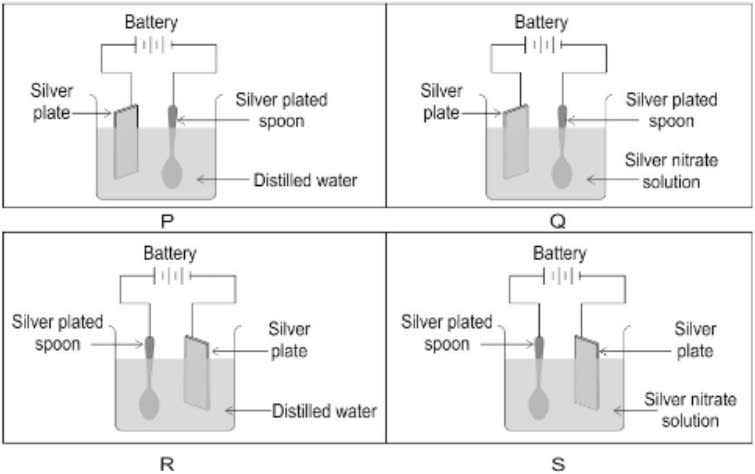1. P
2. Q
3. R
4. S

Q

Reason — Anode should be the plate of silver and cathode should be the spoon to be electroplated. Electrolyte used should be silver nitrate.

#### Question 1(vii)

The basicity of acetic acid is:

1. 1
2. 2
3. 3
4. 4

1

Reason — Acetic acid has four hydrogen atoms in it but it ionises in aqueous solution to produce one hydrogen ion per molecule of the acid. Hence, basicity of acetic acid is 1.

#### Question 1(viii)

A ⟶ A+3; B ⟶ B-1
Number of electrons present in the outermost shell of atoms A and B respectively are:

1. 5, 1
2. 3, 1
3. 3, 7
4. 5, 7

3, 7

Reason — A has donated three electrons from its outer most shell and has +3 charge. B had 7 electrons and has taken one electrons and has -1 charge.

#### Question 1(ix)

A ............... solution is observed after placing Magnesium metal in a solution of Copper sulphate for half an hour.

1. Blue
2. Colourless
3. Reddish brown
4. Dirty green

Colourless

Reason — According to the reactivity series of metals, magnesium is more reactive compared to copper. Hence, it replaces copper from CuSO4 and the blue colour fades as colourless magnesium sulphate is formed and brown bits of copper metal form a precipitate:

Mg + CuSO4 ⟶ MgSO4 + Cu

#### Question 1(x)

An element with atomic no. ............... will form an acidic oxide.

1. 3
2. 17
3. 11
4. 13

17

Reason — Element with atomic number 17 has electronic configuration [2, 8, 7] and hence has 7 electrons in the valence shell. It is a non-metal and will form an acidic oxide.

#### Question 1(xi)

Which of the following is NOT true with respect to nitric acid?

1. It is a strong reducing agent
2. It is a strong oxidizing agent
3. It is unstable to heat
4. It liberates sulphur dioxide gas when treated with potassium sulphite

It is a strong reducing agent

Reason — Nitric acid is a strong oxidizing agent and not reducing agent.

#### Question 1(xii)

................ is the functional group in methanol.

1. >C=O
2. –OH
3. –CHO
4. –COOH

-OH is the functional group in methanol

Reason — The functional group present in methanol [CH3OH] is alcohol [-OH].

#### Question 1(xiii)

The process of electrolysis is an example of:

1. Oxidation reaction
2. Reduction reaction
3. Redox reaction
4. Displacement reaction

Redox reaction

Reason — During electrolysis, the reaction at the cathode involves reduction of cations as they gain electrons to become neutral atoms while that at anode involves oxidation of anions as they lose electrons to become neutral. As redox reactions are reactions where oxidation and reduction takes place simultaneously. Hence, electrolysis is an example of redox reaction.

#### Question 1(xiv)

The catalyst used in Ostwald’s process is ............... .

1. Finely divided iron
2. Graphite
4. Platinum

The catalyst used in Ostwald’s process is platinum.

Reason — Ostwald’s process :

$4\text{NH}_3 + 5\text{O}_2 \xrightarrow[700-800 \degree\text{C}]{\text{Pt}} 4\text{NO} + 6\text{H}_2\text{O} + 21.5 \text{K cals}$

#### Question 1(xv)

An element belongs to third period and sixteenth group. It will have ............... electrons in its valence shell.

1. 2
2. 5
3. 6
4. 3

6

Reason — The element in third period and sixteenth group will have atomic number 16 and electronic configuration 2, 8, 6. Hence, it has 6 valence electrons.

#### Question 2(i)

The setup shown below is that of the fountain experiment with hydrogen chloride gas in the flask.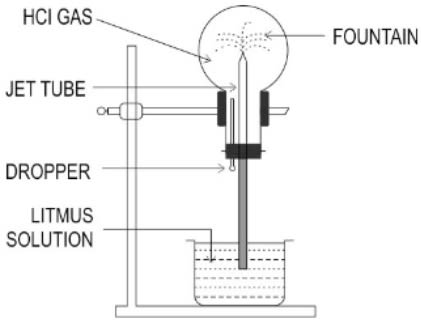The fountain starts when a few drops of water from the dropper are introduced into the flask. Instead of the drops of water, Pooja started the fountain by introducing a few drops of Sodium hydroxide into the flask.

(a) Explain why the litmus solution gets sucked up when Sodium hydroxide is used.

(b) What will be the colour of the fountain when Sodium hydroxide is used? Justify your answer.

(c) If instead of HCl gas, ammonia gas is filled in the flask and water is introduced from the dropper, will there be a different observation? Justify your answer.

When sodium hydroxide is introduced into the flask, it undergoes a neutralization reaction with HCl to form salt and water:

HCl + NaOH ⟶ H2O + NaCl

As HCl is used up in the reaction, this lowers the pressure inside the flask. The outside pressure being higher pushes the litmus solution inside, through the jet tube.

(b) The colour of the litmus solution will remain unchanged so the fountain will be blue in colour.
HCl and sodium hydroxide undergo neutralization reaction to form salt and water which are neutral products. Hence, colour of litmus solution will not change.

(c) No the observation will be the same. Ammonia gas and water produce ammonium hydroxide which is basic in nature, hence again a blue colour fountain will be seen.

#### Question 2(ii)

Match the following Column A with Column B.

Column AColumn B
(a) Aluminium1. Covalent compound
(b) Sulphuric acid2. Carbonate ore
(c) Calcination3. Hall Heroult's process
(d) Calcium Chloride4. Contact Process
(e) Carbon tetrachloride5. Electrovalent compound

Column AColumn B
(a) Aluminium3. Hall Heroult's process
(b) Sulphuric acid4. Contact Process
(c) Calcination2. Carbonate ore
(d) Calcium Chloride5. Electrovalent compound
(e) Carbon tetrachloride1. Covalent compound

#### Question 2(iii)

Complete the following by choosing the correct answers from the bracket:

(a) If an element has one electron in the outermost shell then it is likely to have the ................ [smallest/ largest] atomic size amongst all the elements in the same period.

(b) ................ [sulphuric acid/ hydrochloric acid] does not form an acid salt.

(c) A ................ [reddish brown/ dirty green] coloured precipitate is formed when ammonium hydroxide is added to a solution of ferric chloride.

(d) Alkanes undergo ................ [addition/ substitution] reactions.

(e) An ................ [alkaline/acidic] solution will turn methyl orange solution pink.

(a) If an element has one electron in the outermost shell then it is likely to have the largest atomic size amongst all the elements in the same period.

(b) Hydrochloric acid does not form an acid salt.

(c) A reddish brown coloured precipitate is formed when ammonium hydroxide is added to a solution of ferric chloride.

(d) Alkanes undergo substitution reactions.

(e) An acidic solution will turn methyl orange solution pink.

#### Question 2(iv)

Identify the following:

(a) A bond formed between two atoms by sharing of a pair of electrons, with both electrons being provided by the same atom.

(b) A salt formed by the complete neutralization of an acid by a base.

(c) A reaction in which the hydrogen of an alkane is replaced by a halogen.

(d) The energy required to remove an electron from a neutral gaseous atom.

(e) A homogenous mixture of two or more metals or a metal and a non-metal in a definite proportion in their molten state.

(a) Co-ordinate bond

(b) Normal salt

(c) Halogenation

(d) Ionisation energy

(e) Alloy

#### Question 2(v)

(a) Draw the structural diagram for the following compounds:

1. 1-propanal
2. 1, 2 dichloro ethane
3. But-2-ene

(b) Give the IUPAC name of the following organic compounds: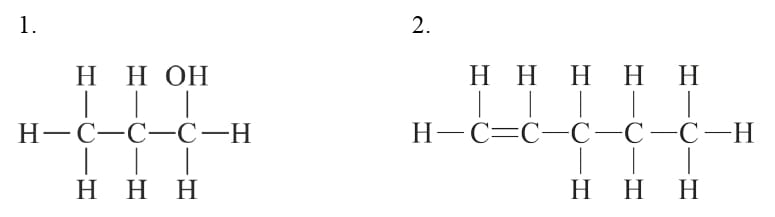(a) Structural diagrams are as follows:

1. 1- propanal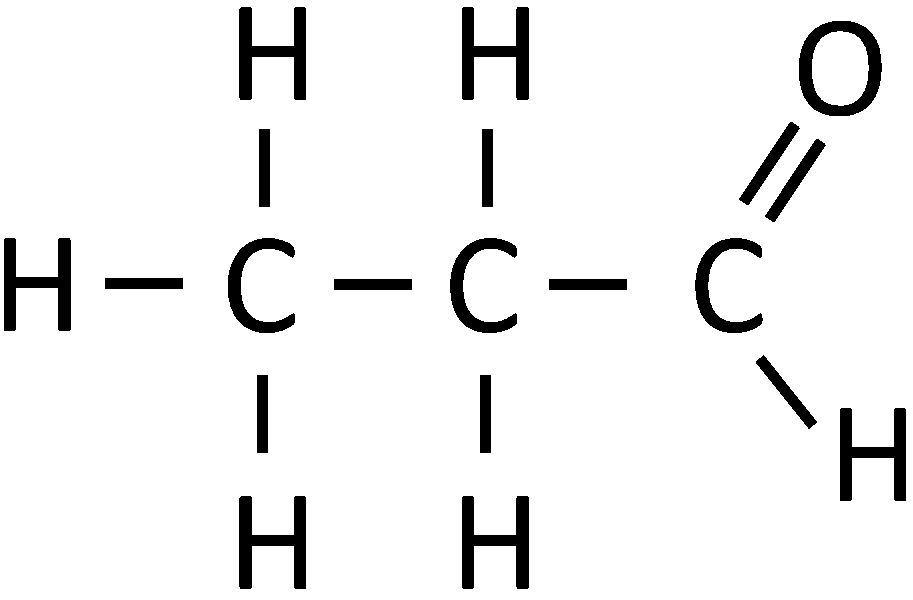2. 1, 2 dichloro ethane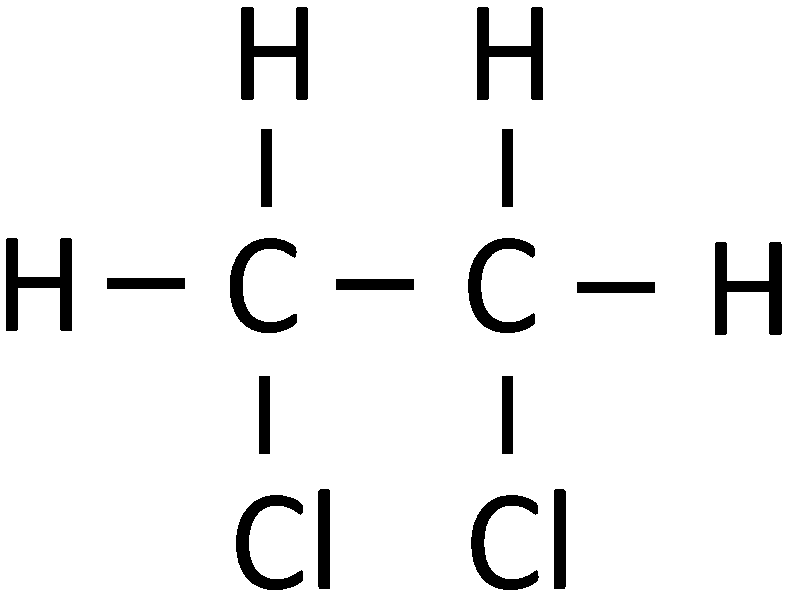3. But-2-ene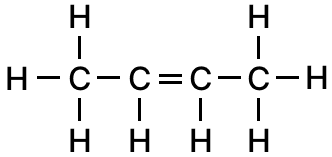(b) IUPAC name are as follows:

1. 1-propanol

2. 1-pentene

## SECTION B (40 Marks)

#### Question 3(i)

Identify the reactant and write the balanced equation for the following:

Nitric acid reacts with compound Q to give a salt Ca(NO3)2, water and carbon dioxide.

Calcium carbonate

CaCO3 + 2HNO3 [dil.] ⟶ Ca(NO3)2 + H2O + CO2

#### Question 3(ii)

What property of Sulphuric acid is exhibited in each of the following cases:

(a) In the preparation of HCl gas when it reacts with Sodium chloride.

(b) When conc. Sulphuric acid reacts with Copper to produce Sulphur dioxide gas.

(a) Non-volatile nature of Sulphuric acid.

(b) Oxidising property of concentrated Sulphuric acid.

#### Question 3(iii)

The electron affinity of an element X is greater than that of element Y.

(a) How is the oxidising power of X likely to compare with that of Y?

(b) How is the electronegativity of X likely to compare with that of Y?

(c) State whether X is likely to be placed to the left or to the right of Y in the periodic table?

(a) Oxidising power of X > Y. Elements with high electron affinity accept electrons more easily hence have greater oxidising power.

(b) Electronegativity of X > Y. Element with high electron affinity has high electronegativity.

(c) X is to the right side of Y as electron affinity increases from left to right in a period.

#### Question 3(iv)

(a) State wether the following statements are TRUE or FALSE. Justify your answer.

1. In an electrovalent compound, the cation attains the electronic configuration of the noble gas that comes after it in the periodic table.

2. In the formation of a compound PQ2, atom P gives one electron to each atom of Q. The compound PQ2 is a good conductor of electricity.

(b) Calculate the number of moles in 22 grams of carbon dioxide.

(a)

1. False
In an electrovalent compound, the cation attains the electronic configuration of the noble gas that comes before it in the periodic table.
2. True
In the formation of a compound PQ2, atom P gives one electron to each atom of Q. The compound PQ2 is a good conductor of electricity in molten or aqueous solution state.

(b) Gram molecular mass of CO2 = 12 + 2(16)
= 12 + 32 = 44 g

As,

44 g of CO2 = 1 mole
∴ 22 g of CO2 = $\dfrac{1}{44}$ x 22 = 0.5 moles

Hence, number of moles in 22 grams of carbon dioxide = 0.5

#### Question 4(i)

The following questions relate to the extraction of Aluminium by electrolysis.

(a) Name the other aluminum containing compound added to alumina.

(b) Give a balanced equation for the reaction that takes place at the cathode.

(a) The compound is cryolite [Na3AlF6].

(b) 2Al3+ + 6e- ⟶ 2Al

#### Question 4(ii)

A gas cylinder of capacity 40 dm3 is filled with gas X the mass of which is 20 g. When the same cylinder is filled with hydrogen gas at the same temperature and pressure the mass of hydrogen is 2 g. Find the relative molecular mass of the gas.

Vapour density of gas =

$\dfrac{\text{Wt. of certain volume of gas }}{\text {Wt. of same volume of H}_2} \\[0.5em] = \dfrac{20}{2} \\[0.5em] = 10 \text{ g}$

Molecular weight = 2 x Vapour density
= 2 x 10 = 20 g

Hence, relative molecular mass of gas is 20 g

#### Question 4(iii)

Give balanced equations for each of the following:

(a) Action of warm water on Aluminium nitride.

(b) Oxidation of carbon with conc. Nitric acid.

(c) Dehydration of ethanol by conc. Sulphuric acid at a temperature of 170°C.

(a) AlN + 3H2O ⟶ Al(OH)3 + NH3 [g]

(b) C + 4HNO3 ⟶ CO2 + 2H2O + 4NO2

(c) C2H5OH $\xrightarrow[170\degree\text{C}]{\text{Conc. H}_2\text{SO}_4}$ C2H4↑ + H2O

#### Question 4(iv)

With respect to Haber’s process answer the following:

(a) Temperature of the reaction

(b) Catalyst used

(c) Balanced equation for the reaction occurring

(a) Temperature : 450-500°C [Optimum temperature]

(b) Catalyst : Finely divided iron [Fe]

(c) N2 + 3H2 ⇌ 2NH3 + Δ

#### Question 5(i)

(a) Ranjana wants to prove that ammonia is a reducing agent. To demonstrate this, she passes ammonia gas over heated copper oxide. What will she observe?

(b) Write a balanced chemical equation for the above reaction.

(a) Black Copper [II] oxide is reduced to reddish brown copper by Ammonia.

(b) 2NH3 + 3CuO ⟶ 3Cu + 3H2O + N2

#### Question 5(ii)

Name the alloy which is made up of:

(a) Copper, Zinc and Tin

(a) Bronze

(b) Solder

#### Question 5(iii)

Seema takes a blue crystalline salt P in a test tube. On heating it produces a white anhydrous powder. P is dissolved in water. Zinc is added to one part of the solution and to another part of the solution Barium chloride is added.

(a) Name the compound P.

(b) Mention one observation when zinc is added to the solution of P.

(c) State the colour of the precipitate formed when barium chloride is added to the solution of P.

(a) P is blue vitriol [CuSO4.5H2O]

(b) Blue colour slowly fades as ZnSO4 is formed.

(c) White ppt. of BaSO4 is obtained.
CuSO4 + BaCl2 ⟶ BaSO4 ↓ + CuCl2

#### Question 5(iv)

Give reasons:

(b) Hydrocarbons can be used as fuels.

(c) Hydrogen chloride gas cannot be collected over water.

(a) In case of ethene, the valencies of atleast 2 carbon atoms are not fully satisfied by hydrogen atoms. The availability of electrons in the double bond makes them more reactive and hence they undergo addition reactions only.

(b) Hydrocarbons are highly combustible and they produce good amount of heat hence they are used as a fuel.

(c) Hydrogen chloride gas is not collected over water since it is highly soluble in water.

#### Question 6(i)

Name the following:

(a) The ore of Zinc containing its sulphide.

(b) The most commonly used oxide ore of Aluminium.

(a) Zinc blende [ZnS]

(b) Bauxite [Al2O3]

#### Question 6(ii)

State one observation in the following cases:

(a) Sodium chloride solution is added to a solution of lead nitrate.

(b) Barium chloride solution is added to a solution of Zinc sulphate.

(a) When sodium chloride is added to a solution of lead nitrate, an insoluble white precipitate of lead chloride is formed.

2NaCl + Pb(NO3)2 ⟶ 2NaNO3 + PbCl2

(b) When barium chloride solution is added to a solution of Zinc sulphate, white ppt. of barium sulphate is obtained which is insoluble in dil. HCl or nitric acid.

ZnSO4 + BaCl2 ⟶ BaSO4 ↓ [white ppt.] + ZnCl2

#### Question 6(iii)

Copper sulphate solution is electrolysed using copper electrodes.

(a) Which electrode [cathode or anode] is the oxidizing electrode? Why?

(b) Write the equation for the reaction occurring at the above electrode.

(a) Anode is the oxidizing electrode. At anode, anions lose electrons and are oxidized.

(b) Cu - 2e- ⟶ Cu2+

#### Question 6(iv)

X [2, 8, 7] and Y [2, 8, 2] are two elements. Using this information complete the following:

(a) ............... is the metallic element.

(b) Metal atoms tend to have a maximum of ............... electrons in the outermost shell.

(c) ............... is the reducing agent.

(a) Y is the metallic element.

(b) Metal atoms tend to have a maximum of three electrons in the outermost shell.

(c) Y is the reducing agent.

#### Question 7(i)

The empirical formula of an organic compound is C3H4N. Its molecular weight is 108. Find the amount of carbon in one mole of the compound. Show all the steps involved.

(Atomic weights: C- 12; H- 1; N- 14)

Empirical formula is C3H4N

Molecular weight = 108

Amount of carbon in 1 mole of the compound = molar mass of carbon x number of carbon atoms in the empirical formula.

= 12 x 3 = 36 g/mol

Hence, in 1 mole of the compound C3H4N, there is approximately 36 grams of carbon.

#### Question 7(ii)

(a) Mahesh prepared a basic solution X that has a pH 7. How will the pH of the solution X change on addition of the following:

1. Hydrochloric acid

2. a solution of a base

(b) The atomic number of an element is 15. To which group will this element belong to?

(a) 1. On adding the acid, pH value will decrease.

1. On adding the solution of a base, pH value will increase.

(b) As the atomic number is 15, so the electronic configuration will be 2, 8, 5. Hence, it will belong to VA group.

#### Question 7(iii)

8.2 grams of calcium nitrate is decomposed by heating according to the equation

2Ca(NO3)2 ⟶ 2CaO + 4NO2 + O2

Calculate the following:

(a) Volume of nitrogen dioxide obtained at STP

(b) Mass of CaO formed

[Atomic weights: Ca –40 , N—14, O—16]

$\begin{matrix} 2\text{Ca}(\text{NO}_3)_2 & \longrightarrow & 2\text{CaO} & + & 4\text{NO}_2 & + & \text{O}_2 \\ 2[(40) + & & 2(40 & & 4[22.4] \\ 2(14 +3(16))] & & + 16) & & \text{ lit.} \\ = 328 \text{ g} & & = 112 \text{g} \\ \end{matrix}$

(i) 328 g of Ca(NO3)2 produces 4(22.4) lit of nitrogen dioxide.

∴ 8.2 g of Ca(NO3)2 will produce $\dfrac{4 \times 22.4}{328}$ x 8.2 = 2.24 lit of nitrogen dioxide.

Hence, vol of nitrogen dioxide evolved = 2.24 lit.

(ii) 328 g of Ca(NO3)2 produces 112 g of calcium oxide.

∴ 8.2 g of Ca(NO3)2 will produce $\dfrac{112}{328}$ x 8.2 = 2.8 g of calcium oxide.

Hence, 2.8 g of calcium oxide is produced.

#### Question 8(i)

State giving reasons if:

(a) zinc and aluminium can be distinguished by heating the metal powder with concentrated sodium hydroxide solution.

(b) calcium nitrate and lead nitrate can be distinguished by adding ammonium hydroxide solution to the salt solution.

(a) Zinc and aluminium cannot be distinguished by heating the metal powder with concentrated sodium hydroxide solution as they both react with conc. alkalis to form soluble sodium salts and hydrogen gas.

Zinc reacts to form sodium zincate

Zn + 2NaOH ⟶ H2 + Na2ZnO2 [sodium zincate]

Aluminium reacts to form sodium aluminate

2Al + 2NaOH + 2H2O ⟶ 3H2 + 2NaAlO2 [sodium aluminate]

(b) Yes, calcium nitrate and lead nitrate can be distinguished using ammonium hydroxide solution. Ammonium hydroxide on reaction with lead nitrate gives chalky white precipitate of Pb(OH)2. No precipitation occurs on adding Ammonium hydroxide to calcium nitrate even when it is added in excess.

Pb(NO3)2 + 2NH4OH ⟶ Pb(OH)2 + 2NH4NO3

#### Question 8(ii)

Draw the electron dot diagram of Hydronium ion.

Electron dot diagram showing the structure of Hydronium ion is given below: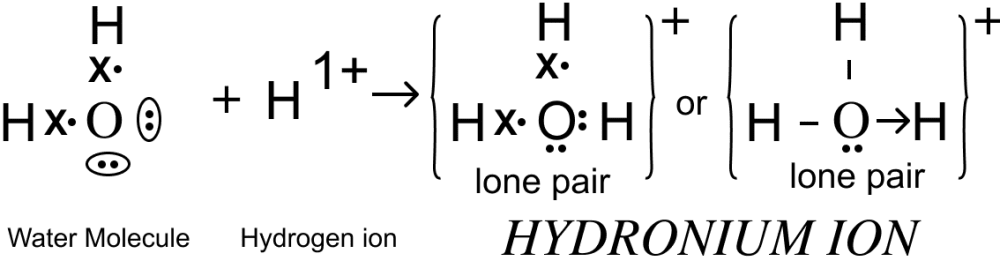#### Question 8(iii)

Give balanced equations for the following:

(a) Laboratory preparation of ethyne from calcium carbide.

(b) Conversion of acetic acid to ethyl acetate.

(c) Laboratory preparation of nitric acid.

(a) $\underset{\text{calcium carbide}}{\text{CaC}_2} + \underset{\text{water}}{2\text{H}_2\text{O}} \longrightarrow \underset{\text{ethyne}}{\text{C}_2\text{H}_2} + \underset{\text{calcium hydroxide}}{\text{Ca(OH)}_2}$

(b) $\underset{\text{ethanol}}{\text{C}_2\text{H}_5\text{OH}} + \underset{\text{acetic acid}}{\text{CH}_3\text{COOH}} \xrightarrow[\Delta]{\text{Conc. H}_2\text{SO}_4} \underset{\text{ethyl acetate}}{\text{CH}_3-\text{COO}-\text{C}_2\text{H}_5} + \text{H}_2\text{O}$

(c) $\underset{[\text{conc.}]}{\text{KNO}_3} + \underset{[\text{conc.}]}{\text{H}_2\text{SO}_4} \xrightarrow{\lt 200 \degree\text{C}} \underset{[\text{acid salt}]}{\text{KHSO}_4} + \text{HNO}_3$

#### Question 8(iv)

Identify the following substances:

(a) An alkaline gas which produces dense white fumes when reacted with HCl gas.

(b) The anion present in the salt, which produces a gas with the smell of rotten eggs when reacted with dil. HCl.

(c) The particles present in strong electrolytes.